### Home > CCA2 > Chapter Ch11 > Lesson 11.3.1 > Problem11-95

11-95.
1. Sketch a graph, f(x), that has the number and types of roots described for each situation below. Homework Help ✎

1. 5 real roots

2. 3 real and 2 complex roots

3. 4 complex roots

4. 4 complex and 2 real roots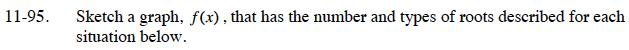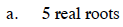Since the degree is an odd number, it will start and end in different directions.
A real root occurs when the polynomial intersects the x-axis, so this graph should intersect the x-axis 5 times.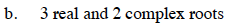This polynomial has 5 roots, but only 3 x-intercepts.

A possible graph would be a polynomial with 3 x-intercepts plus another bend.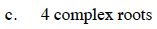The degree of this polynomial is even, so it starts and ends in the same direction.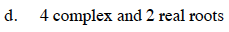See part (a), (b), and (c).